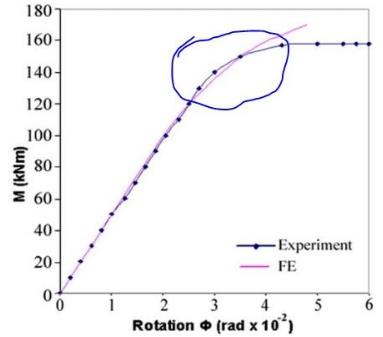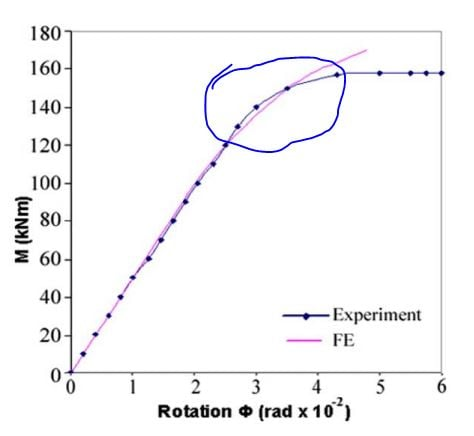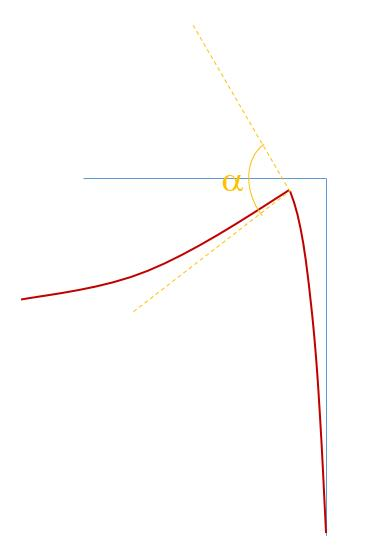# Getting the Moment that the beam transfer to the column, modelling with solids - PORTIC

Hello.

I would like to learn how to calculate the moment which the beam transfer to the column, becasue the connection is semi rigid, so, part of the moment is transfered, and the other not, because the beam rotate.

The objective is to plot a graph Moment X Rotation. The rotation is easy to calculate: I measure the vertical displacement, so divide by the length of the beam and it is ok. But the moment I do not know how to calculate. I think that maybe with the bolt stress, or something like that.

Can anybody help me?

The model is attached.

• I gave this answer before. Maybe someone else can provide some guidance.

• edited August 2018

Thank you Peter. Your answer is the case that I said, the beam transfer full moment to the column.

On the other hand, modeling all the components of the connection, beam, endplate, bolts, and column. It is not true that full moment (100%) is transfred from the beam to the column, because in mostly the connection is semi rigid. So I would like to know this %%% that is not transfered to the column, because this %%% of moment sta in the beam, but appear as a rotation, did you understand?

I am looking for some commands as Sandeep said, but I did not find.

• I thought your goal was to make the moment vs rotation plot that is published in the paper you provided.In that paper on bolted joint simulation, I found the definition of rotation and the model was a simple load P at the end of a cantilever of length L so the moment M = PL.  As the end load ramps up to P over time, the rotation builds up. The graph of M vs Rotation begins as linear, then curves over as plasticity effects begin in the joint.  But the formula for moment doesn't change.  It is always PL, where P increases from zero to a large value over the course of a simulation. There is no change in the definition of moment as the joint begins to have significant distortion.

The difference between the paper and your model is that instead of an end load P on a cantilever, you have a distributed load Q on a beam supported at both ends. The link in my post above shows how to calculate M for that configuration.  So in a similar fashion, as the load Q increases from zero to a large value over the course of a simulation, you can plot M vs Rotation.

Do you agree that you can make a similar plot to the one in the paper with this information?

• edited August 2018

Yes, I agree with you.

But I do not agree with the paper I provided. Because parts of the moment is dissipated as rotation. But if I do not find anything about it. I will use this formulation.

• edited August 2018

Fabricio,

The paper is actually right. Just think about the equilibrium of the beam alone, without the other components (column and joint).

As static equilibrium equations must be fullfiled, the moment in the support of a cantiliver beam subjected to a concentrated force P in the end, no matter whether it is a fully or partially rigid support, will always be PL. This 'dissipated' moment that you are thinking of is actually an increase in rotation, not a reduction in moment, you see?

In your model, you have a beam that is supported by two columns which have rigid ends and, therefore, your model is statically indeterminate and the solution is not as simple as calculating PL, but you can retrieve reactions from ansys after the solution and get the full static determination of the structural frame and then perform the same procedure.

Also, you mentioned that you are getting rotation from just dividing the vertical displacement in midspan by the half-length of the beam. This is actually imprecise. Remember both the beam and the column will deform and the actual joint rotation will be the difference between the final angle between them (alpha in the attached figure, which is a little larger than 90º ) and the initial one (90º in your case). Also remember that both the beam and the column rotations must be taken locally at the joint and not in the end of the beam as the rotation decreases towards the center of the beam or the support of the column that you are studying.• edited August 2018

Thank you João. I will take the reactions and compare. Maybe the same.

Yes, for the rotation I have to subtract the column rotation. This is dZ/Lbeam - dx/Lcolumn.

Thank you!!

• edited August 2018

Fabricio, remember that dz/Lbeam will be a simplified estimate of the rotation as it varies along the span of the beam and the column. Perhaps taking a node that is closer to the joint will lead to more precise results.